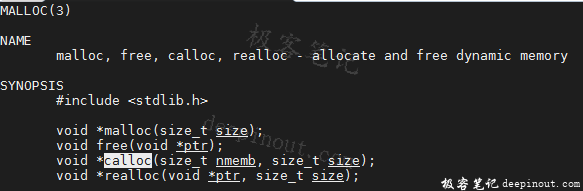# C库函数 calloc申请零值内存

C 库函数 void *calloc(size_t nitems, size_t size) 分配所需的内存空间，并返回一个指向它的指针。malloccalloc 之间的不同点是，malloc 不会设置内存为零，而 calloc 会设置分配的内存为零。

## calloc函数说明``````void *calloc(size_t nitems, size_t size);
``````

• nitems - 要被分配的元素个数。
• size - 元素的大小。

## calloc函数实例

``````#include <stdio.h>
#include <stdlib.h>

int main()
{
int i, n = 3;
int *a;

a = (int*)calloc(n, sizeof(int));
for( i=0 ; i < n ; i++ ) {
printf("%d\n",a[i]);
}
free (a);
return(0);
}
``````

``````0
0
0
``````

• 微信订阅
• 回顶